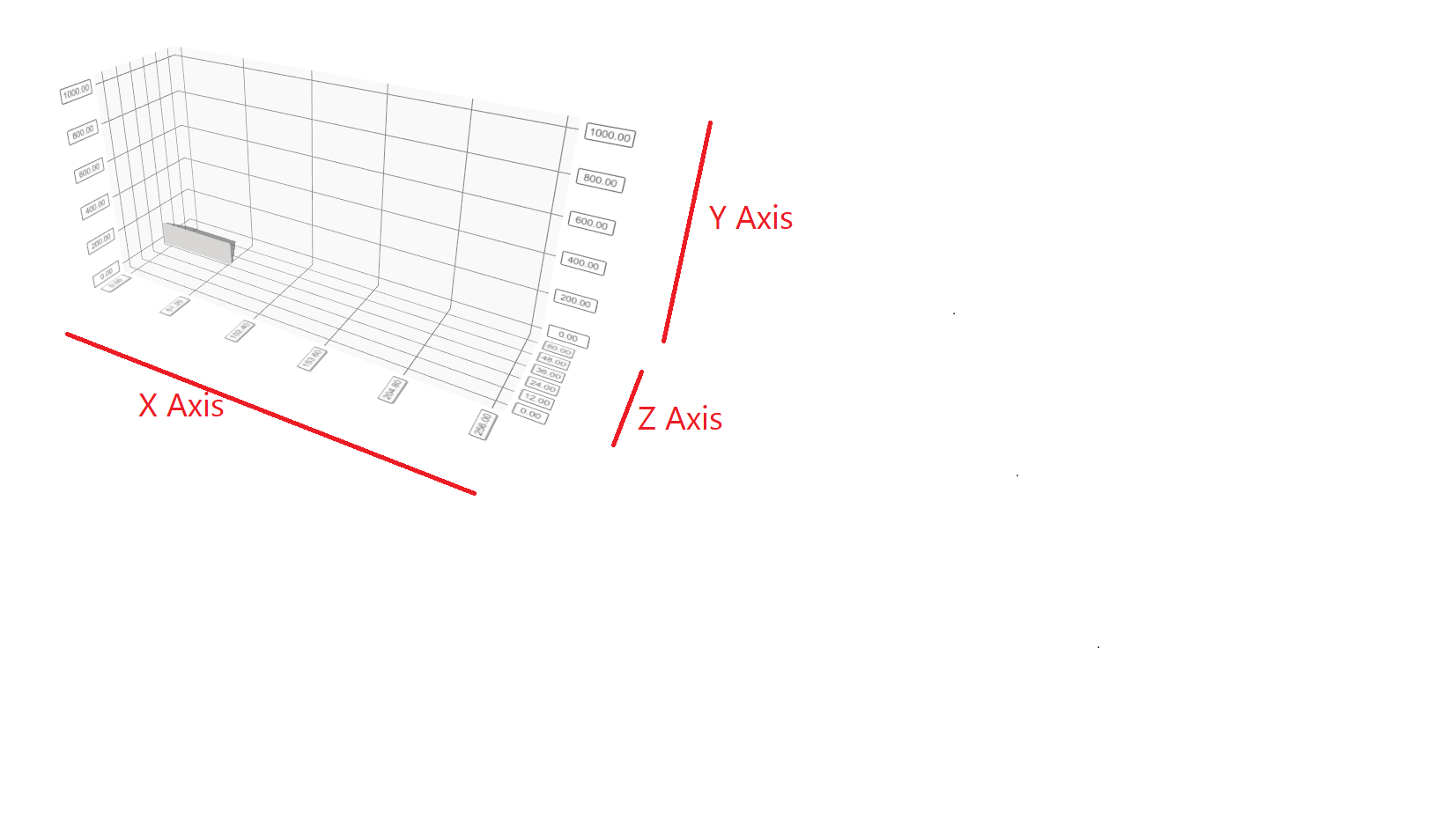# I have a question about 3d graph.

• I used Q3dSurface... I wonder that

Problem 1.

The value of the y-axis set from 0 to 255, but the value shown wants to be seen as a value of 0 to 360.I think the following code would do, but the value shown is marked "255 * 1.40625".

Q3DSurface *graph = new Q3DSurface();
graph ->axisY()->setLabelFormat ("%d * 1.40625")

The result I want is to make the value of the Y-axis appear 0 to 360, not 0 to 256.

I would appreciate it if you could tell me which function to use to shown on the Y axis.

Problem 2.

I would like to create a 3d graph with a range of X values from 0 to 60, a range of Y values from 0 to 360, and a range of Z values from 0 to 1000.

As shown in the image above, the width of the y-axis is shown to be quite small.

Please let me know how to make a 3d graph of the same length on the X, y, and Z axes.